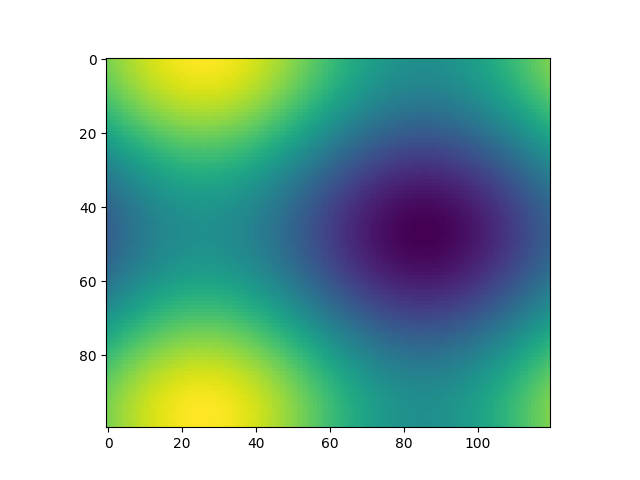Animated image using a precomputed list of images¶import numpy as np
import matplotlib.pyplot as plt
import matplotlib.animation as animation

fig, ax = plt.subplots()

def f(x, y):
return np.sin(x) + np.cos(y)

x = np.linspace(0, 2 * np.pi, 120)
y = np.linspace(0, 2 * np.pi, 100).reshape(-1, 1)

# ims is a list of lists, each row is a list of artists to draw in the
# current frame; here we are just animating one artist, the image, in
# each frame
ims = []
for i in range(60):
x += np.pi / 15.
y += np.pi / 20.
im = ax.imshow(f(x, y), animated=True)
if i == 0:
ax.imshow(f(x, y))  # show an initial one first
ims.append([im])

ani = animation.ArtistAnimation(fig, ims, interval=50, blit=True,
repeat_delay=1000)

# To save the animation, use e.g.
#
# ani.save("movie.mp4")
#
# or
#
# writer = animation.FFMpegWriter(